Email:

# What Does A Resistor Do?

## What is a resistor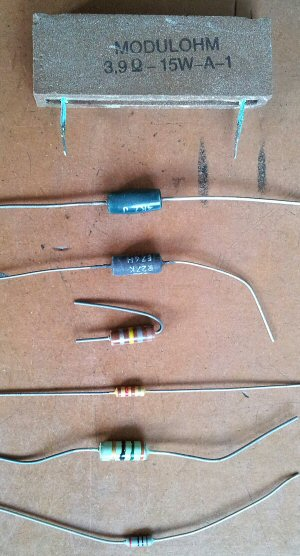A collection of resistors
from my junk box

### Ohms and Mhos

Resistance is measured in Ohms using the greek symbol called Omega Ω. It is the exact opposite to conductance which is measured in Mhos using an inverted Omega ℧ as a symbol. I have always found that little fact very funny for some reason but it is true.

If you have no idea what resistance is all about then it might be easier for me to explain what conductance is first. If something is said to have a high conductance then it will allow for a lot of electricity, or current to flow. High conductance means it conducts a lot.

Resistance is the opposite to conductance so a high resistance means that it will conduct only a little. By varying the values of resistors and their positions in a circuit we can control the current flow in that circuit.

Resistors are generaly specified by their resistance values in Ohms but they all come with a secondary value which tells us how much power they can dissipate before they become too hot and burn up. This is important because all resistors that have a current flowing in them will get hot to some extent as some of the electrical energy is converted to heat inside the resistor. If it gets too hot then it will melt or catch fire which is not a good thing.

For low power circuits you might only need resistors rated as low as one quarter of a Watt or 250mW. The smallest of the resistors from my junk box in the image are 250mW. For devices that involve high voltages and/or current then you may need to use bigger, more robust resistors. The large resistor at the top of the picture is a 15W type. This kind of resistor might be found in power supplies or high power amplifiers etc.

One of the most important principles of basic electronics tells us that the voltage (V), current (I), resistance (R) and power (P) within any circuit are related and can therefore be calculated. In the case of resistors the formula is very simple and it is called Ohms Law. It is named after the German physicist Georg Ohm.

### Ohm's Law

V = I * R

That's it. I told you it was simple. All you have to remember is that the voltage between any two points in a circuit can be calculated by multiplying the current flowing and the resistance between the two points.

### Power dissipation

The power dissipated by a circuit can be calculated by multiplying the voltage across the circuit by the current flowing.

P = I * V

By combining the formulas above we can determine the power in any circuit in several ways from the formulae below. You are free to use whichever formula fits with the measurement of voltage, current and resistance that you have available.

• P = I * V
• P = I2 * R
• P = V2 / R

### Resistor color code chart

Small resistors generally have little or no space available to write out their value on the body. To overcome this problem you will see that many resistors have bands of color around the body. The position and color of the bands tell us both the resistance value and the precision that the resistor is manufactured to.

Color coded resistors will generally have 4 bands of color with higher precision resistors using 5 bands. The first 3 or 4 bands hold the resistance value and the last band gives the precision. There should be a larger gap between the resistance bands and the tolerance which indicates which end of the resistor to read the colors from.

R Band
Color123MulTol
±%
Black0001
Brown111101
Red2221002
Orange3331K
Yellow44410K
Green555100K0.5
Blue6661M0.25
Violet77710M0.10
Grey888 0.05
White999
Gold    ±5
Silver    ±10

The first 2 (or 3) bands of resistance value give us the first 2 (or 3) digits of resistance. The 3rd (or 4th) band provides a multiplier. For instance a resistor with resistance code Yellow-Violet-Red would be 47 * 100 (red is 2 so add 2 zeros). This would be a 4700 Ω or 4.7k Ω resistor. This can also be written as 4k7 Ω

Now subscribe to our newsletter and don't miss a thing

* Required information
(will not be published)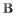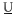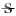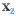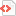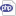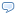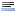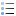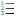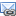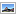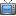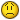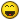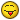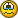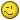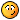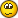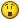Enter code:

Notify me of new comments via email.

Remember my form details on this computer.